# 换元积分法

"换元积分法" 是求积分的方法，适用于可以写成一个特定格式的函数。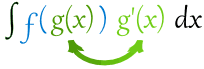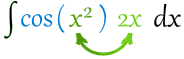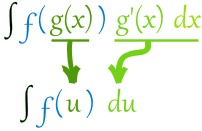### 例子：∫cos(x2) 2x dx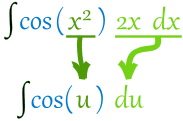cos(u) du = sin(u) + C

u=x2 代回去：

sin(x2) + C

### 例子：∫cos(x2) 6x dx

cos(x2) 6x dx = 3cos(x2) 2x dx

（常数乘数可以移到外面。见 积分法则。）

3cos(u) du = 3 sin(u) + C

u=x2 代回去：

3 sin(x2) + C

### 例子：∫x/(x2+1) dx

x/(x2+1) dx = ½2x/(x2+1) dx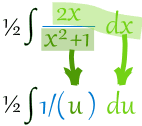½1/u du = ½ ln(u) + C

u=x2+1 代回去：

½ ln(x2+1) + C

### 例子：∫(x+1)3 dx

…… x+1 的导数是 …… 1！

(x+1)3 dx = (x+1)3 · 1 dx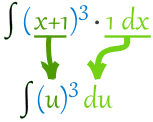u3 du = (u4)/4 + C

u=x+1 代回去：

(x+1)4 /4 + C

## 总结